# Association submodel

Uses the Simile “association submodel” to specify a relationship between objects (e.g. ownership of fields by farmers)

## Land-use dynamics - demonstration model

ModelId:
landuse1
SimileVersion:
3.1+

This model illustrates one approach to modeling land-use dynamics. In this case, individal units of land ("patches") can be in one of two states: forest or crop. Each patch can switch from one state to the other, depending on various factors including the current state of the forest or crop in the patch.

Results:

The following sequence of screen shots shows how the cropping area on the left (yellow) gradually encroaches into the forest. It is then replaced by young forest (light green), until eventually it in turn is cut down and the cycle continues.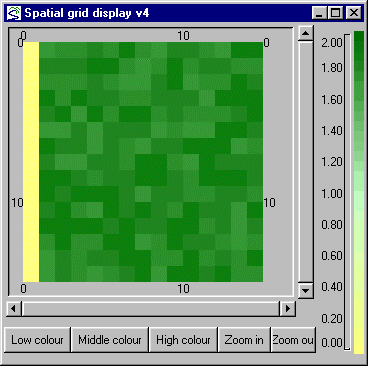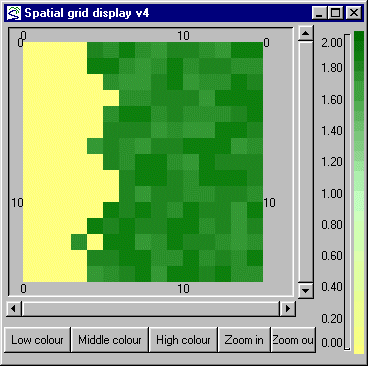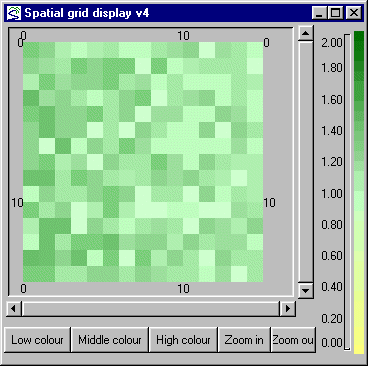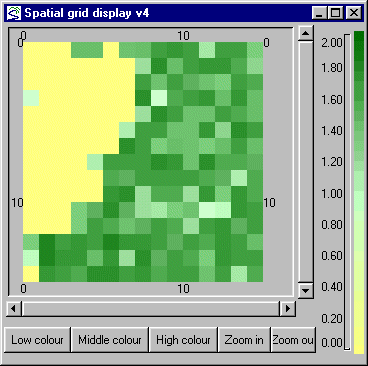## A hierarchy of hexagonal tilings

ModelId:
hex_levels
SimileVersion:
4.0

This model has been included to illustrate a number of techniques used to create complex structures in which model components can interact. It allows an area to be represented as a series of hexagonal tilings, with increasing levels of detail. Each hexagon in one level corresponds to a group of 7 hexagons in the next level down. The arrangement is based on that used in the HOOFS model (S. P. Oom et al, 2004) It would be straightforward to implement this layout with a series of nested submodels, each representing one layer of the tiling.

Equations:

Equations in top level Variable magic magic = sqrt(3) Variable offa_even offa_even = [ 0.5, -0.5,-1, -0.5, 0.5,1] Variable offa_odd offa_odd = cos(theta)*[offa_even]-sin(theta)*[offb_even] Variable offb_even offb_even = [ 0.866, 0.866,0, -0.866, -0.866,0] Variable offb_odd offb_odd = sin(theta)*[offa_even]+cos(theta)*[offb_even] Variable theta theta = atan(3*sqrt(3)) Equations in hexagon Variable centre x centre x = if posn_in_parent==0 then hi_cx_lower else hi_cx_lower+magic*element(element([[offb]],my_level),int(posn_in_parent)) Where: my_level=my level posn_in_parent=posn in parent [[offb]]= ../level info/offb {hi_cx_higher}= ../hierarchy/hi_cx (to hexagon in higher) hi_cx_lower= ../hierarchy/hi_cx (to hexagon in lower) magic= ../magic Variable centre y centre y = if posn_in_parent==0 then hi_cy_lower else hi_cy_lower-magic*element(element([[offa]],my_level),int(posn_in_parent)) Where: my_level=my level posn_in_parent=posn in parent [[offa]]= ../level info/offa {hi_cy_higher}= ../hierarchy/hi_cy (to hexagon in higher) hi_cy_lower= ../hierarchy/hi_cy (to hexagon in lower) magic= ../magic Variable my level my level = hi_level_lower+1 Where: {hi_level_higher}= ../hierarchy/hi_level (to hexagon in higher) hi_level_lower= ../hierarchy/hi_level (to hexagon in lower) Variable my parent my parent = if index(1)==1 then 1 else int((index(1)+5)/7) Variable posn in parent posn in parent = if index(1)==1 then 0 else int(fmod(index(1)-2,7)) Equations in hierarchy Condition cond1 cond1 = index(1) is my_parent_lower Where: my_parent_lower= ../hexagon/my parent (from hexagon in lower) my_parent_higher= ../hexagon/my parent (from hexagon in higher) Variable dummy dummy = index(2)==1 Variable hi_cx hi_cx = if dummy then 0 else centre_x_higher Where: centre_x_lower= ../hexagon/centre x (from hexagon in lower) centre_x_higher= ../hexagon/centre x (from hexagon in higher) Variable hi_cy hi_cy = if dummy then 0 else centre_y_higher Where: centre_y_lower= ../hexagon/centre y (from hexagon in lower) centre_y_higher= ../hexagon/centre y (from hexagon in higher) Variable hi_level hi_level = if dummy then 0 else my_level_higher Where: my_level_lower= ../hexagon/my level (from hexagon in lower) my_level_higher= ../hexagon/my level (from hexagon in higher) Equations in level info Variable even level even level = fmod(index(1),2)== 1.0 Variable offa offa = side*(if even_level then [offa_even] else [offa_odd]) Where: even_level=even level [offa_even]= ../offa_even [offa_odd]= ../offa_odd Variable offb offb = side*(if even_level then [offb_even] else [offb_odd]) Where: even_level=even level [offb_even]= ../offb_even [offb_odd]= ../offb_odd Variable side side = 50/7^((index(1)-1)/2) Equations in for display Condition cond1 cond1 = my_level==6 Where: my_level= ../my level Variable colour colour = index(1) Variable xpts xpts = centre_x+element([[offa]],my_level) Where: centre_x= ../centre x [[offa]]= ../../level info/offa my_level= ../my level Variable ypts ypts = centre_y+element([[offb]],my_level) Where: centre_y= ../centre y [[offb]]= ../../level info/offb my_level= ../my level

Results:

Here is the diagram of the hexagons at the lowest level: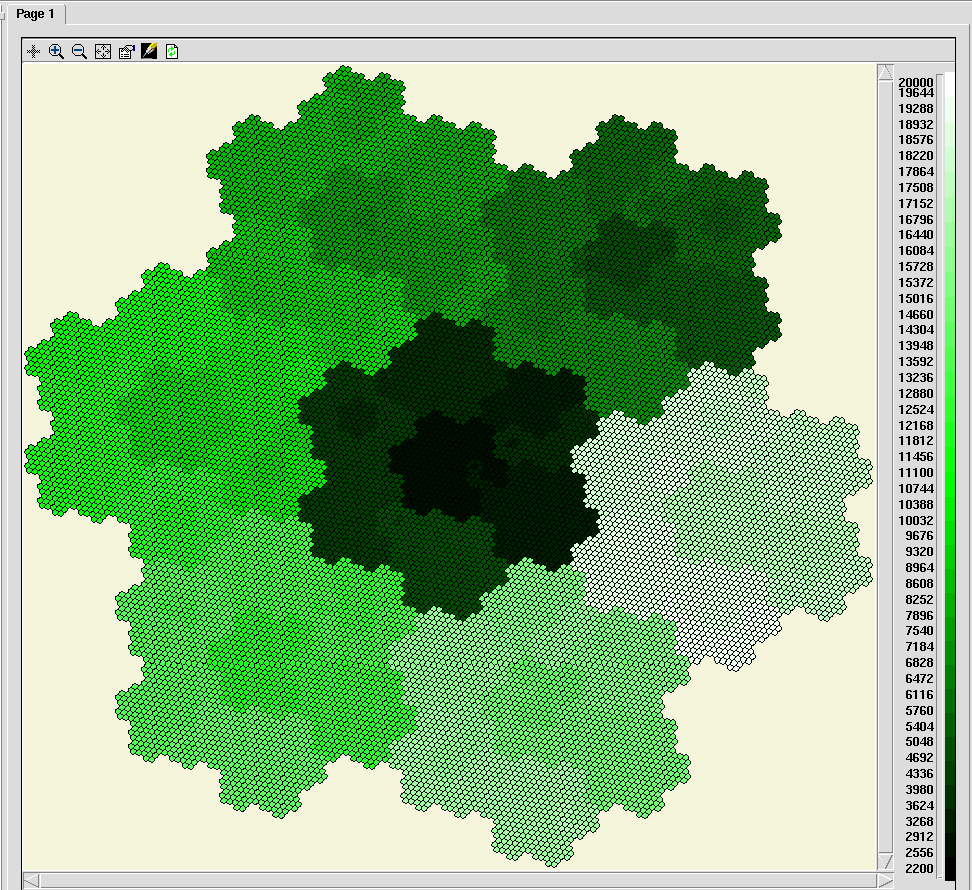References:

S.P. Oom, J.A. Beecham, C.J. Legg and A.J. Hester (2004) Foraging in a complex environment: from foraging strategies to emergent spatial properties     http://www.sciencedirect.com/science/article/pii/S1476945X04000522

## Food-web modelling: two trophic layers

ModelId:
feeding1
SimileVersion:
3.1+

This model simulates the feeding relationships between two trophic layers: plants and herbivores. Each trophic layer is represented in terms of a number of species, and we model the feeding between (potentially) each species at one level and each species on the other level. An association submodel is used to specify which species of herbivore actually feeds on which species of plant.## Embryo morphogenesis

ModelId:
embryo1
SimileVersion:
3.1+

This model implements ideas developed by John Gurdon, Cambridge University, on the role of activin in morphogenesis. The following gives a biological statement about the system on the left, and the corresponding Simile realisation on the right.

Results:

The animation below shows the activin concentration on the left; the SMAD concentrations in the middle, and the resulting cell commitment to cell type on the right.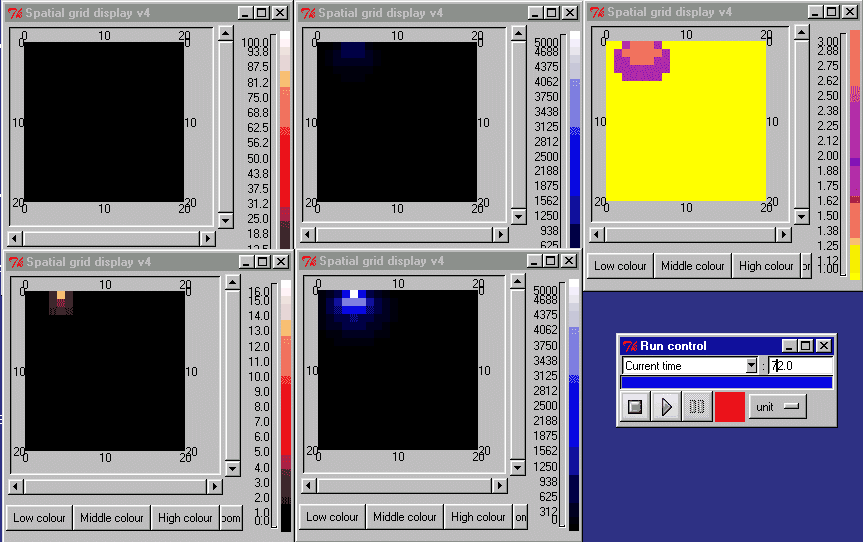## Individual particle based diffusion model

ModelId:
diffusion
SimileVersion:
diffusion

This models molecules in a lattice. They are held in their lattice positions by mutual repulsion. It starts with a low vibrational energy which gradually increases. They are divided into two types for display purposes to illustrate diffusion. To start with they vibrate around their intital positions, then occasionally exchange positions until above a certain energy the fixed positions are lost and they all get mixed.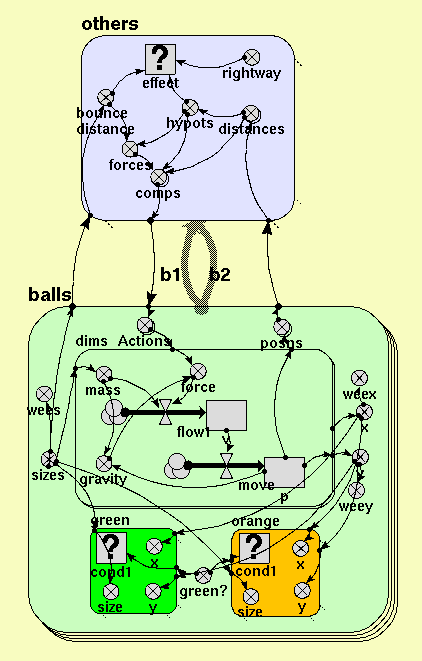Equations:

Contained submodel(s)
others
balls

Submodel others

Submodel others is a relation submodel for a relation between balls and itself.

Condition for existence of submodel

effect

Units: boolean
effect = hypots<bounce_distance and rightway
Where:
bounce_distance is the variable bounce distance in this submodel.
hypots is the variable hypots in this submodel.
rightway is the variable rightway in this submodel.

Variable(s)

comps

Units: array(1,2)
comps = forces*[distances]/hypots
Where:
forces is the variable forces in this submodel.
hypots is the variable hypots in this submodel.
[distances] is the variable distances in this submodel.

bounce distance

Units: 1
bounce distance = sizes_b1+sizes_b2
Where:
sizes_b1 is the variable sizes in balls
sizes_b2 is the variable sizes in balls

distances

Units: array(1,2)
distances = [posns_b1]-[posns_b2]
Where:
[posns_b1] is the variable posns in balls
[posns_b2] is the variable posns in balls

hypots

Units: 1
hypots = hypot(element([distances],1),element([distances],2))
Where:
[distances] is the variable distances in this submodel.

forces

Units: 1
forces = pow(bounce_distance/hypots,4)
Where:
bounce_distance is the variable bounce distance in this submodel.
hypots is the variable hypots in this submodel.

rightway

Units: boolean
rightway = index(1)<index(2)

Submodel balls

Submodel balls is a fixed membership submodel with 32 members.

Variable(s)

Actions

Units: array(1,2)
Actions = sum({[comps_b1]})-sum({[comps_b2]})
Where:
{[comps_b1]} is the variable comps in others
{[comps_b2]} is the variable comps in others

green?

any(index(1)==[2,3,4,5,7,8,9])
Units: boolean
green? = any(index(1)==[2,3,4,5,7,8,9,12,13,14,17,18,22,23,27,32])

posns

Units: array(1,2)
posns = [p]
Where:
[p] is the compartment p in balls/dims

x

Units: 1
x = element([p],1)
Where:
[p] is the compartment p in balls/dims

y

Units: 1
y = element([p],2)
Where:
[p] is the compartment p in balls/dims

sizes

Units: 1
sizes = 15

wees

Units: 1
wees = sizes/10
Where:
sizes is the variable sizes in this submodel.

weex

Units: 1
weex = x/10+45
Where:
x is the variable x in this submodel.

weey

Units: 1
weey = y/10+45
Where:
y is the variable y in this submodel.

Contained submodel(s)
green
dims
orange

Submodel balls/green

Submodel green is a conditional submodel.

Condition for existence of submodel

cond1

Units: boolean
cond1 = green_
Where:
green_ is the variable green? in balls

Variable(s)

size

Units: 1
size = sizes
Where:
sizes is the variable sizes in balls

x

Units: 1
x = x
Where:
x is the variable x in balls

y

Units: 1
y = y
Where:
y is the variable y in balls

Submodel balls/dims

Submodel dims is a fixed membership submodel with 2 members.

Flow(s)

move
Units: 1
move = v
Where:
v is the compartment
v in this submodel.

flow1
Units: 1
flow1 = force/mass
Where:
force is the variable
force in this submodel.
mass is the variable mass in this submodel.

Variable(s)

gravity

Units: 1
gravity = 10*mass*if p<0 then 0-p elseif p>100 then 100-p else 0
Where:
mass is the variable mass in this submodel.
p is the compartment p in this submodel.

force

Units: 1
force = a+element([Actions],index(1))+rand_var(-10,10)

mass

Units: 1
mass = sizes*sizes/100
Where:
sizes is the variable sizes in balls

Submodel balls/orange

Submodel orange is a conditional submodel.

Condition for existence of submodel

cond1

Units:
cond1 = missing

Variable(s)

size

Units: 1
size = sizes
Where:
sizes is the variable sizes in balls

x

Units: 1
x = x
Where:
x is the variable x in balls

y

Units: 1
y = y
Where:

Results:

These images show the positions of particles at the start and end of the run.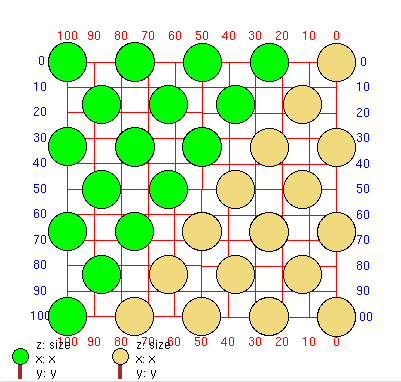## Banker/broker/consumer multi-agent model

ModelId:
dave1
SimileVersion:
3.1+

This model was developed in relation to the SLIE project (Sustainable Lifecycles for Information Ecosystems). That project is based on agent-based approaches: this model aims to explore the extent to which such models can be implemented in the Simile environment.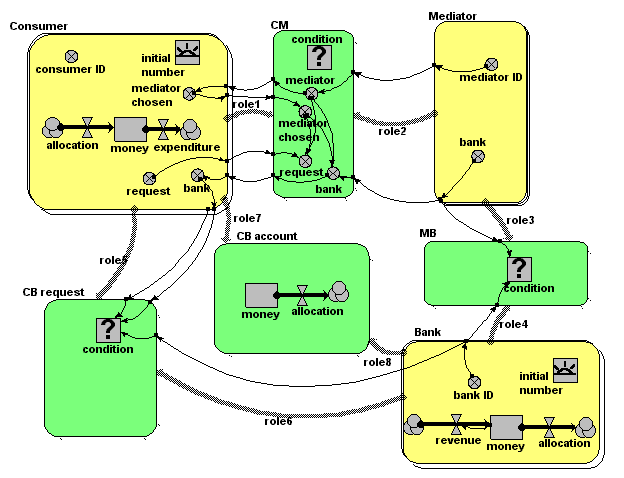Equations:

Equations in submodel Bank

creation: initial number = 2
compartment: money = 100000
flow: revenue = 0.1*money
flow: allocation = 0
variable: bank ID = index(1)

Equations in submodel Consumer
creation: initial number = 4
compartment: money = 0
flow: allocation = 0
flow: expenditure = 100
variable: consumer ID = index(1)
variable: request = (if rand_var(0,1)<0.2 then 1 else 0)
variable: mediator chosen = greatest({mediator})
variable: bank = sum({bank})

Equations in submodel Mediator
variable: mediator ID = index(1)
variable: bank = -1*floor(rand(1,2.9999))

Equations in submodel CM
condition: condition = (rand_const(0,1)<0.5)
variable: request = (if mediator==mediator_chosen then request else 0)
variable: mediator = mediator_id
variable: mediator chosen = mediator_chosen [in submodel Consumer]
variable: bank = (if mediator==mediator_chosen then bank else 0)

Equations in submodel MB
condition: condition = (bank==bank_id)

Equations in submodel CB request
condition: condition = (bank==bank_id&&request>0)

Equations in submodel CB account
compartment: money = 0

## Data-specified association between instances of the same submodel

ModelId:
data_specified_assoc2
SimileVersion:
soc2 Simile version :

This model demonstrates how to set up an association between submodel instances using a data file containing pairs of instances.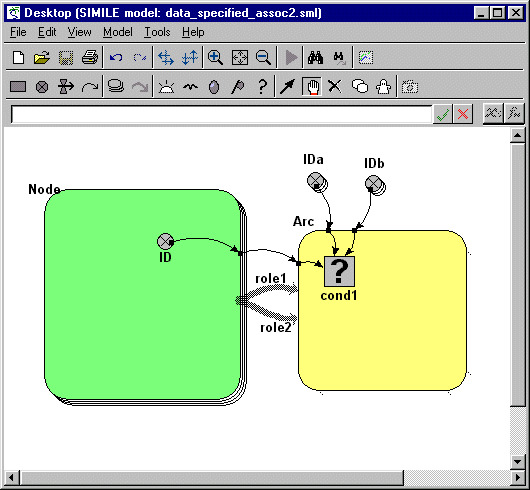Category:
Technique
Equations:

Equations in ../Desktop

IDa = [1,2,3]
IDb = [2,3,4]

Equations in Node
ID = index(1)

Equations in Arc
cond1 = (any(ID == [IDa]and ID_0 == [IDb])or any(ID == [IDb]and ID_0 == [IDa]))
where
ID = ../Node/ID (from Node in role1)
ID_0 = ../Node/ID (from Node in role2)
[IDa] = ../IDa

Model tags:

## Data-specified association between two different submodels

ModelId:
data_specified_assoc1
SimileVersion:
3.1+

This model demonstrates how to set up an association between submodel instances using a data file containing pairs of instances.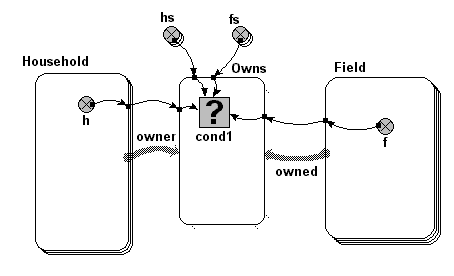Equations:

Equations in ../Desktop

hs = [1,1,2]
fs = [3,4,4]
Comment: This two arrays together specify that household 1 owns fields
3 and 4, while household 2 owns field 4 (part ownership allowed!)

Equations in Household
h = index(1)

Equations in Owns
cond1 = any(var1 == [hs]and f == [fs])
where:
var1 = ../Household/h (from Household in owner)
f = ../Field/f (from Field in owned)
[hs] = ../hs
[fs] = ../fs

Equations in Field
variable:f = index(1)

## Representing links between a number of countries, using an association submodel

ModelId:
SimileVersion:
3.1+

This model is an example of setting up an association between objects using a data file. The file consists of pairs of IDs, with each pair specifying one instnace of the association. In this case, we specify which countries share a trading association (i.e. which country can export to another country). The data file which specifies the country trading pairs also contains information on the volume of trade between each country pair. This is totalled for both the exporting country and the importing country.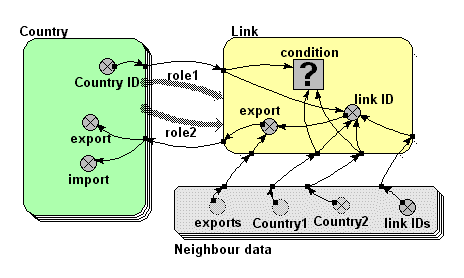Model tags:

## Skeleton cohort model

ModelId:
cohort1
SimileVersion:
5.97

This example shows how to implement a basic cohort model in Simile.

A cohort model is one way of modelling a population. The individuals are grouped into cohorts, typically on the basis that they were born at the same time. Each cohort has as a minimum a variable representing the number of individuals in that cohort, along with possibly other cohort-specific attributes, such as the average size of the individuals in the cohort.

Equations:

Variable: n_total = sum({n})

Where: {n} = Cohort/n

Variable: population_total_size = sum({cohort_total_size})
Where: {cohort_total_size} = Cohort/cohort_total_size

--------------------------------------------------------------
Submodel Bigger

Submodel "Bigger" is an association submodel between "Cohort" and itself with roles "other" and "me".

Condition: condition = other_size>me_size
Where:
me_size = Value(s) of ../Cohort/size from submodel "Cohort" in role "me"
other_size = Value(s) of ../Cohort/size from submodel "Cohort" in role "other"

Variable: total_size_of_bigger_cohort = other_cohort_total_size
Where:
me_cohort_total_size = Value(s) of ../Cohort/cohort_total_size from submodel "Cohort" in role "me"
other_cohort_total_size = Value(s) of ../Cohort/cohort_total_size from submodel "Cohort" in role "other"

-------------------------------------------------------------------------
Submodel Cohort

Submodel "Cohort" is a population submodel.

Compartment: n
Initial value = 100
Rate of change = - loss

Compartment: size
Initial value = 0
Rate of change = + growth

Flow: growth = gr*(1-size/max_size)

Flow: loss = 5

Immigration: create_new_cohort = 1

Loss: remove_cohort = n<=0

Variable: cohort_total_size = n*size

Variable: gr = 1.5

Variable: max_size = 10

Variable: total_size_of_all_bigger_cohorts = sum({cohort_total_size_of_bigger_me})
Where:
{cohort_total_size_of_bigger_me} = ../Bigger/total_size_of_bigger_cohort for submodel "Cohort" in role "me"

Results:

The results shown here illustrate the basic functioning of this model.

The following figure shows a set of panels, each one showing the values for a specified variable at time 10. These were made using Simile's 'snapshot' tool, and enable us to trace the values as they are processed in the model.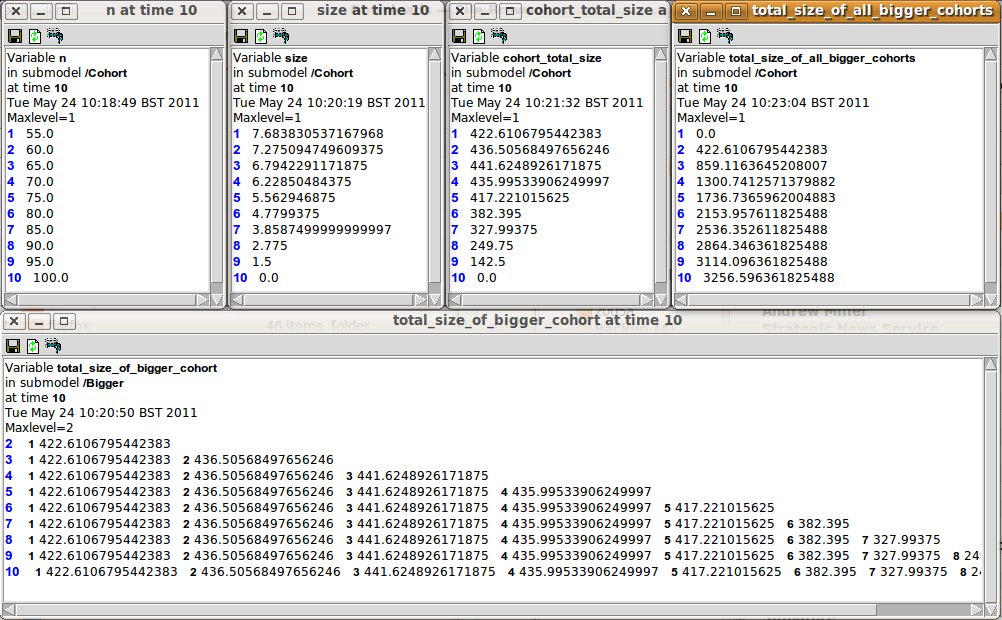The first 3 panels on the top row show, for each of the 10 cohorts created up to this time, the number of tress (n) and the size of the average tree (size) in the cohort, along with the total size (n x size). You can see that the number of tress declines in a cohort declines by 5 each time step, and that the average tree in a cohort grows at a reducing rate as the cohort gets older.

The bottom window shows the total_size values for the individual cohorts that are bigger than the specified cohort. Thus, cohort 2 only has one value (since there is only one bigger than it is), while cohort 3 has 2 values, and so on.

The top-right window shows 'total_size_of_all_bigger_cohorts' - the sum (along a row) for al the total_size values of bigger cohorts for a particular cohort. This is the key value which enables the impact of bigger cohorts on the growth of a cohort to be modelled.

The graph below shows how the 'total_size_of_all_bigger_cohorts' changes over time, for each cohort. The lowest curve is for the second cohort (since the first cohort has nothing bigger than it. Each successive cohort starts off at a higher value (since the total size of cohorts above it is increasing), until we get to the point where each newly-created cohort starts off with the same total_size above it, which decreases as that cohort in turn increases in size.## Pages